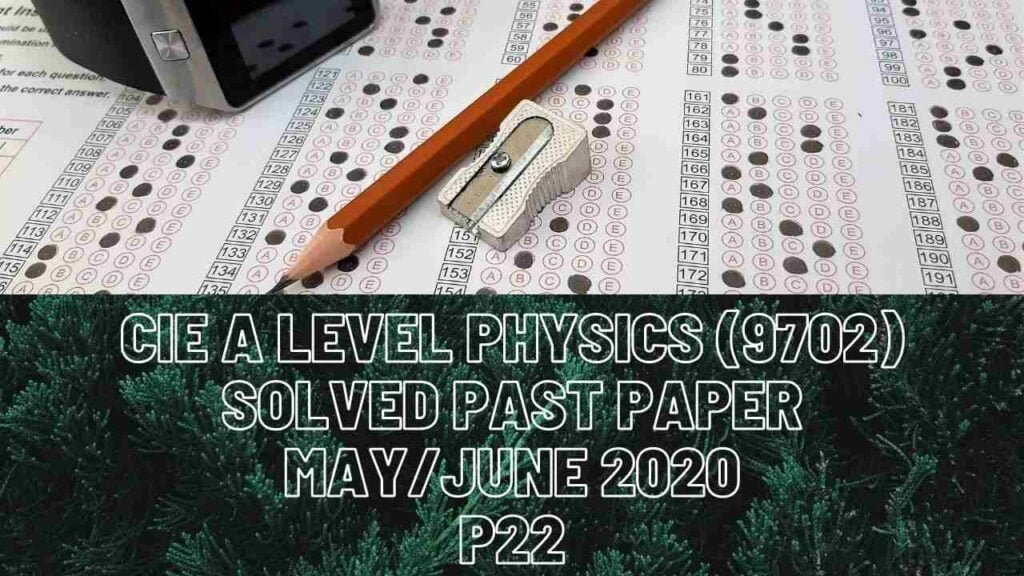A Level Physics### CIE A Level Physics Solved Past Paper May/June 2020 P22

1c) Since the car is moving constant speed, its driving force must be equal to drag force acting on the car. To calculate the speed with which this car is moving can be calculated by using the formula output power=driving force*velocity where driving force is equal to drag force in this Q.

2 bi) The ball hits the wall when it completes its vertical motion and that finishes when final velocity of that ball reaches zero. Acceleration of the ball would be -9.81m/s^2 as its moving against gravity and initial vertical velocity is vertical component of initial velocity at angle to horizontal 15m/s.

ii) During horizontal component of a projectile motion, its speed remains the same because no acceleration takes place in that direction thus no resultant force.

iii) The horizontal velocity remains constant throughout and is horizontal component of 15m/s of velocity.

3 bi) At highest point, the ball had 321.4J of energy in the form of g.p.e and when hits the ground, it was possessing 111.1J of energy in the form of kinetic energy. There is a loss in energy which must have been due to air resistance meaning that work done by drag force is 321.4-111.1=210.3J so resistive force must be work done by that resistive force/distance that ball travels. Resistive force=210.3/78=2.7N

c) When the ball starts moving towards the ground, air resistance starts to get greater, and as a result, resultant force starts to decrease which leads to a decrease in acceleration too and a time comes when both air resistance and ball’s weight get equal. At that time, there is no acceleration and the ball is now travelling at terminal velocity.

4 bi) One complete wavelength is distance between 2 successive crests or troughs. 0.48m of length has 2 successive troughs and a crest too so that makes 1 complete and a half wave.

6 ai) Whenever a battery has its internal resistance, e.m.f of battery won’t be equal to p.d across circuit because some volts are lost.

bii) Vout=(Vin*R1)/(R1+R2) where R1 is the resistance of a component or combination of components across which you are calculating voltage, Vin is the e.m.f of battery and R2 is the resistance that is in series with R1. In fig.6.2, if you calculate the voltage across the stand-alone resistor then that’s Vout, Vin is 9.0V, R1 is the resistance of that resistor and R2 is the combined resistance of the 2 resistors in parallel connection.